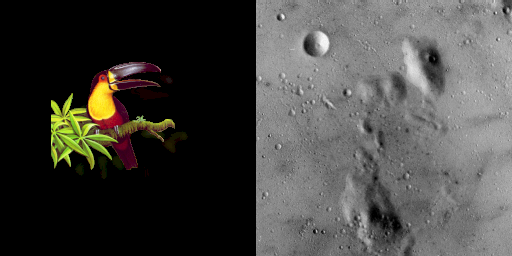## StackViews.jl

No more 🐱🐱
Author JuliaArrays
Popularity
5 Stars
Updated Last
2 Years Ago
Started In
April 2021

# StackViews

`StackViews` provides only one array type: `StackView`. There are multiple ways to understand `StackView`:

• inverse of `eachslice`
• `cat` variant
• view object
• lazy version of `repeat` special case

## `StackView` as the inverse of `eachslice`

`StackView` can be seen as the inverse of `eachslice`:

```julia> using StackViews

julia> X = rand(100, 100);

julia> StackView(collect(eachslice(X, dims=1)), 1) == X
true```

## `StackView` as a variant of `cat`

`StackView` works very similar to `cat` and its `vcat`/`hcat`/`hvcat` variants in Base.

```julia> using StackViews

julia> A = reshape(collect(1:6), 2, 3)
2×3 Matrix{Int64}:
1  3  5
2  4  6

julia> B = reshape(collect(7:12), 2, 3)
2×3 Matrix{Int64}:
7   9  11
8  10  12

julia> StackView(A, B; dims=3) # mostly equivalent to `cat(A, B, dims=3)`
2×3×2 StackView{Int64, 3, 3, ...}:
[:, :, 1] =
1  3  5
2  4  6

[:, :, 2] =
7   9  11
8  10  12```

Unlike `cat`s, `StackView` always creats new dimension:

```julia> StackView(A, B; dims=1) # `cat(A, B, dims=1)` outputs 4×3 Matrix
2×2×3 StackView{Int64, 3, 1, ...}:
[:, :, 1] =
1  2
7  8

[:, :, 2] =
3   4
9  10

[:, :, 3] =
5   6
11  12

julia> StackView(A, B; dims=2) # `cat(A, B, dims=2)` outputs 2×6 Matrix
2×2×3 StackView{Int64, 3, 2, ...}:
[:, :, 1] =
1  7
2  8

[:, :, 2] =
3   9
4  10

[:, :, 3] =
5  11
6  12```

## `StackView` as a view object

Without `StackView`, people use `reshape` + `cat` for the previous examples:

```julia> StackView(A, B, dims=1) == cat(map(x->reshape(x, 1, axes(x)...), (A, B))...; dims=1)
true```

but `StackView` is only a view and thus don't create any memory:

```frames = [rand(1000, 1000) for _ in 1:100];
@btime StackView(\$frames, Val(1)); # 143.905 ns (0 allocations: 0 bytes)
@btime cat(map(x->reshape(x, 1, axes(x)...), \$frames)...; dims=1); # 1.127 s (1119 allocations: 763.06 MiB)```

Of course, since it is a view, if you modify it, original arrays get modified, too:

```A = [1, 2, 3, 4]
B = [5, 6, 7, 8]
sv = StackView(A, B)
fill!(sv, -1) # A and B are modified
A == B == fill(-1, 4) # true```

If indexed in contiguous memeory order, it has almost zero overhead for `getindex` and `setindex!`:

```function arrsum_cart(A::AbstractArray)
rst = zero(eltype(A))
@inbounds for I in CartesianIndices(A)
rst += A[I]
end
return rst
end

As = StackView(frames);
Ac = cat(map(x->reshape(x, axes(x)..., 1), frames)...; dims=3);
As == Ac # true

@btime arrsum_cart(\$As); # 122.703 ms (0 allocations: 0 bytes)
@btime arrsum_cart(\$Ac); # 123.813 ms (0 allocations: 0 bytes)```

## `StackView` as a lazy version of `repeat` special case

`StackView` allows you to stack the same array object multiple times, which makes a special version of `repeat` when there's only one none-1 repeat count:

```A = rand(1000, 1000);
n = 100;
StackView([A for _ in 1:n]) == repeat(A, ntuple(_->1, ndims(A))..., n) # true
@btime StackView([\$A for _ in 1:\$n]); # 403.156 ns (2 allocations: 1.75 KiB)
@btime repeat(\$A, ntuple(_->1, ndims(\$A))..., \$n) # 590.043 ms (4 allocations: 762.94 MiB)```

## More examples

When arrays are of different types and sizes, `StackView` just kills `cat`s:

```julia> using StackViews, PaddedViews

julia> A = collect(reshape(1:8, 2, 4));

julia> B = collect(reshape(9:16, 4, 2));

4×4×2 StackView{Int64, 3, 3, ...}:
[:, :, 1] =
1   3   5   7
2   4   6   8
-1  -1  -1  -1
-1  -1  -1  -1

[:, :, 2] =
9  13  -1  -1
10  14  -1  -1
11  15  -1  -1
12  16  -1  -1

4×4×2 StackView{Int64, 3, 3, ...):
[:, :, 1] =
-1  -1  -1  -1
1   3   5   7
2   4   6   8
-1  -1  -1  -1

[:, :, 2] =
-1   9  13  -1
-1  10  14  -1
-1  11  15  -1
-1  12  16  -1```

There is some mind work here but by chaining more views you can get some interesting result:

```using PaddedViews, StackViews
using ImageCore, ImageShow, TestImages, ColorVectorSpace

toucan = testimage("toucan") # 150×162 RGBA image
moon = testimage("moon") # 256×256 Gray image

# equivalently, you can just use `mosaic(toucan, moon; nrow=1)`